# KSEEB Solutions for Class 8 Maths Chapter 6 Theorems on Triangles Ex 6.3

In this chapter, we provide KSEEB SSLC Class 8 Maths Chapter 6 Theorems on Triangles Ex 6.3 for English medium students, Which will very helpful for every student in their exams. Students can download the latest KSEEB SSLC Class 8 Maths Chapter 6 Theorems on Triangles Ex 6.3 pdf, free KSEEB SSLC Class 8 Maths Chapter 6 Theorems on Triangles Ex 6.3 pdf download. Now you will get step by step solution to each question.

## Karnataka Board Class 8 Maths Chapter 6 Theorems on Triangles Ex 6.3

Question 1.
The exterior angles obtained on producing the base of a triangle both ways are 104° and 136°. Find the angles of the triangle.
In triangle ABC, BC is produced on either sides.
Let ∠ABC = 140° and ∠ACE = 136°
∠ABD +∠ABC = 180° [ Linear pair]
104 + ∠ABC = 180°
∠ABC = 180 – 104
∠ ABC = 76°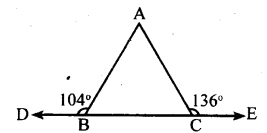∠ACB + ∠ACE = 180° [Linearpair]
∠ACB + 136° = 180°
∠ACB = 180 – 136 ∠ACB = 44°
∠ABD + ∠ACB + ∠BAC = 180°
[Sum of the angles of a triangle 180° ]
76 + 44 + ∠BAC = 180°
120 + ∠BAC = 180°
∠BAC = 180 – 120 ∠BAC = 60°
Three angles are 76°, 44° & 60°

Question 2.
Sides BC, CA and AB of a triangle ABC are produced in order, forming exterior angles ∠ACD, ∠BAE, and∠CBF.
Show that ∠ACD + ∠BAE + ∠CBF = 360°.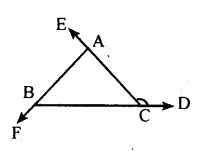ABC + ∠CBF = 180° [Linear pair]………..(i)
∠ACB + ∠ACD = 180° [Linear pair]……(ii)
∠BAC + ∠BAE = 180° [Linear pair]…….(iii)
∠ABC + ∠CBF + ∠ACB + ∠ACD+
∠BAC + ∠BAE = 180 + 180 + 180
∠ABC + ∠ACB + ∠BAC +
∠CBF + ∠ACD + ∠BAE = 540°
180 + ∠CBF + ∠ACD + ∠BAE = 540°
[Sum of the angles of a triangle 180°]
∠CBF + ∠ACD + ∠BAE = 360°

Question 3
Compute the value of x in each of the following figures
i.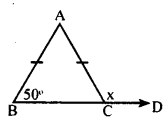AB = AC
∴ ∠ABC = ∠ACB = 50°
∠ACB + ∠ACD = 180° [Linear pair]
50 + x = 180°
x = 180 – 50 = 130°

ii.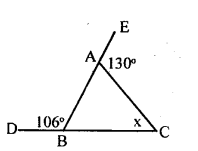∠ABC + ∠CBF = 180° [Linear pair].
106°+∠ABC = 180°
∠ABC = 180 – 106 = 74°
∠EAC = ∠ABC + ∠ACB
130° =74 +x
130 – 74 = x
∴x = 56°

iii.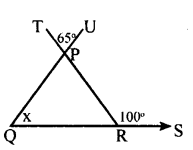∠QPR = ∠TPU = 65°
[Vertically opposite angles]
∠PRS = ∠PQR + ∠QPR [Exterior angle=Sum of interior opposire angle]
100 = 65 + x
100 – 65 = x
35 = x
∴ x = 35°.
iv.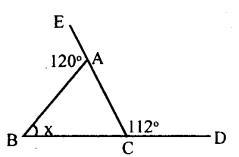∠BAE + ∠BAC = 180° [Linear pair]
120° + ∠BAC = 180°
∠BAC = 180 – 120
∠BAC = 60°
∠ACD = ∠BAC + ∠ABC
[Exterior angle = Sum of interior opposuite angle]
112° = 60+x
112 – 60 = x
52 = x
x = 52°
v.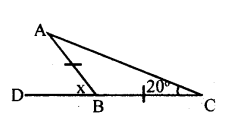In Δ ABC, BA = BC (data)
∴ ∠BAC = ∠BCA = 20°
[Base angles of an isosceles traingle]
∠ABD = ∠BAC + ∠BCA
[Exterior angle=Sum of interior opposite angle]
x = 20 + 20
x=40°

Question 4.
In figure QT ⊥ PR, ∠TOR = 40° and ∠SPR = 30° find ∠TRS and ∠PSQ.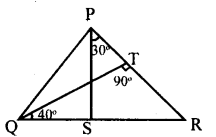In ΔTQR,
∠TQR + ∠QTR + ∠TRQ = 180°
40 + 90 + ∠TRQ = 180°
130 + ∠TRQ = 180
∠ TRQ = 180 – 130
∠ TRQ = 50°
∠ TRS = 50° [∠PRS is same as ∠TRS ]
In Δ PRS, RS is produced to Q
∴Exterior∠PSQ = ∠SPR + ∠PRS = 30 + 50
∠PSQ = 80°

Question 5.
An exterior angle of a triangle is 120° and one of the interior opposite angle is 30 °. Find the other angles of the triangle.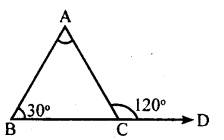In Δ ABC, BC is produced to D. Let
∠ACD = 120° and ∠ABC = 30°
Exterior ∠ACD = ∠BAC + ∠ABC
120 = ∠BAC + 30
120 – 30 = ∠BAC
90° = ∠BAC
∴∠BAC = 90
∠ACB +∠ACD = 180° [Linear pair]
∠ACB + 120 = 180°
∠ACB = 180 – 120
∠ACB = 60°
Other two angles are 90° & 60°

All Chapter KSEEB Solutions For Class 8 maths

—————————————————————————–

All Subject KSEEB Solutions For Class 9

*************************************************

I think you got complete solutions for this chapter. If You have any queries regarding this chapter, please comment on the below section our subject teacher will answer you. We tried our best to give complete solutions so you got good marks in your exam.

If these solutions have helped you, you can also share kseebsolutionsfor.com to your friends.

Best of Luck!!